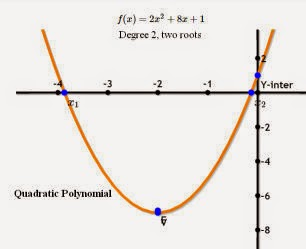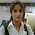Polynomials

Study Points

1. An expression of the form a0 + a1x + a2x2 + ----- + anxn where an is called a polynomial in variable x of degree n. where; a0 ,a1, ----- an are real numbers and are called terms/co-efficients of the polynomial and each power of x is a non negative integer.

2. Polynomials in the variable x are denoted by f(x), g(x), h(x) etc.
e.g. f(x) = a0 + a1x + a2x2 + ----- + anxn

3. A polynomial p(x) = a (where a is constant) is of degree 0 and is called a constant polynomial.

4. A polynomial p(x) = ax + b is of degree 1 and is called a linear polynomial. e.g. 4x - 3, 5x...

5. A polynomial p(x) = ax2 + bx + c of degree 2 and is called a quadratic polynomial.
e.g. 3x2 -x + 5, 1- x2...

6. A polynomial p(x) = ax3 + bx2 + cx + d of degree 3 and is called a cubic polynomial.
e.g. √5x3 - 2x2 + 5x -1...

7. Expressions like  5x2  +  1/xx-2 - x  + 1 are not polynomials

8. Zeroes of a polynomial f(x): A real number α is a zero of the polynomial f(x)
if and only if f(α) = 0. The graph of y = f(α) intersects the X-axis.

9. A linear polynomial has only one zero (at the most).

10. A Quadratic polynonial at the most has two zeroes.

11. A cubic polynomial  at the most has three zeroes.

12. A polynomial may not have real zeroes. f(x) ≠ 0 for any value of x. Hence f(x) has no real zeroes.

13. A polynomial of degree n can have at most n real zeroes.

14. For any quadratic polynomial ax2 + bx + c = 0, a 0, the graph of this equation i.e. y = ax2 + bx + c has one of the two shapes called Parabolas.

15. The parabola for the given quadratic equation will open upwards like ∪ or downwards like ∩ , depending on whether a > 0 or a < 0.

16. If α and β are the zeroes of a quadratic polynomial ax2 + bx + c, then

17. A quadratic polynomial whose zeroes are given by α and β is given by:
p(x) = x2 - (α + β)x + αβ
= x2 - (sum of zeroes)x + product of zeroes.

18. If α, β and γ are zeroes of the cubic polynomial ax3 + bx2 + cx + d then:
α+β+γ = -b/a
αβ + βγ + γα = c/a
αβγ = -d/a

19. The division algorithm states that given any polynomial p(x) and any non-zero polynomial g(x),
there are polynomials q(x) and r(x) such that
p(x) = g(x) q(x) + r(x), where r(x) = 0 or degree r(x) < degree g(x).1 comment:

1.This comment has been removed by the author.